# HBSE 8th Class Maths Solutions Chapter 13 Direct and Inverse Proportions InText Questions

Haryana State Board HBSE 8th Class Maths Solutions Chapter 13 Direct and Inverse Proportions InText Questions and Answers.

## Haryana Board 8th Class Maths Solutions Chapter 13 Direct and Inverse Proportions InText Questions

Try These (Page 204)

Question 1.
Observe the following tables and find if x and y are directly proportional.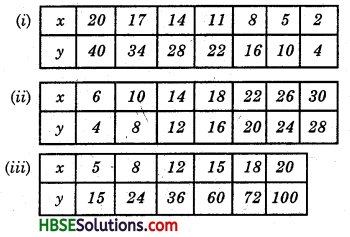Solution:
$$\frac{x}{y}$$ = $$\frac{x_{1}}{y_{1}}$$ = $$\frac{x_{2}}{y_{2}}$$ ………..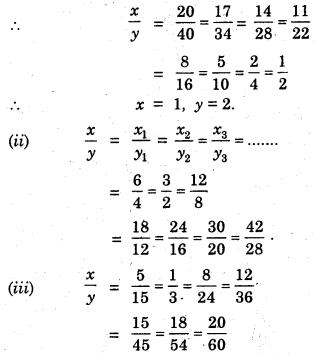Question 2.
Principal = Rs. 1000, Rate = 8% per annum. Fill in the following table and find which type of interest (simple or compound) changes in direct proportion with time period.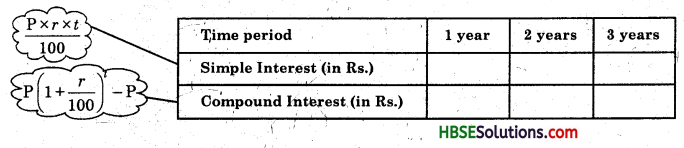Solution: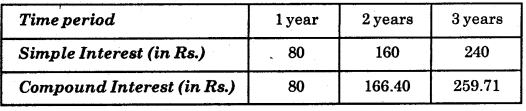Try These (Page 211)

Question 3.
Observe the following tables and find which pair of variables (here x and y) are in inverse proportion.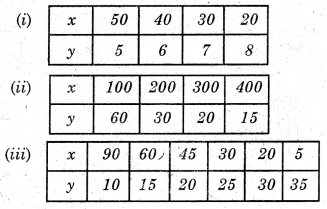Solution:
(i) Two quantities are said to vary inversely if the increase in one quantity causes the decrease in the other quantity.
In this case if y1, y2 are the values of y corresponding to the values x1, x2 of x respectively then
x1y1 = x2y2
$$\frac{x_{1}}{x_{2}}$$ = $$\frac{y_{1}}{y_{2}}$$
x × y = x1y1 = x2y2 = ………
x × y ≠ 50 × 5 ≠ 40 × 6 ≠ 30 × 7 ≠ 20 × 8
Hence, table (i) is not inversely proportional.

(ii) x × y = x1 × y1 = x2 × y2
= x3 × y3…………
= 100 × 60 = 200 × 30
= 300 × 20 = 400 × 15
= 600 = 6000 = 6000 = 6000
Hence table (ii) is inversely proportional.(iii) x × y = x1y1= x2 × y2 = x3 × y3 = ……..
= 90 × 10 = 60 × 15
= 45 × 20 = 30 × 25
= 20 × 30 = 5 × 35
= 900 = 900 = 900 ≠ 750
≠ 600 ≠ 175
Hence, in table (iii) 90, 10; 60, 15; 45, 20 are inversely proportion but other are not inversely proportional.